HLIBpro  1.2
BLAS/LAPACK Interface

The foundation of HLIBpro is provided by the BLAS and LAPACK software packages. Especially the advanced functions of LAPACK, e.g. singular value decomposition, play a crucial role in the H-arithmetics. To simplify the usage of these functions and to reduce the potential for errors, an extra layer was implemented between the H-arithmetics and the BLAS/LAPACK functions. The BLAS module provides this layer.

All data classes in the BLAS namespace share special properties with respect to the memory management of the underlying data:

• Each class is implemented as a template with the base datatype as the template parameter, e.g. double or complex.
• Each memory block, e.g. a vector or matrix data, has a reference counter associated with it. This allows several objects to point to the same memory block, e.g. you may address sub vectors or sub matrices. If all references to a memory block are gone, the memory is automatically freed.
• Vector and matrix objects only store pointers to the actual memory block and are therefore small in terms of memory footprint. This permits efficient return values in functions, e.g. you may return an actual matrix instead of just a pointer to it.
• Data is not necessarily contiguous inside a memory block, e.g. you may address each second element of a memory block. This is especially needed to address sub matrices, where each column starts with a certain offset with respect to the previous column.
• You may not change the size of a vector or matrix, only assigning a vector or matrix of a different size.
• In contrast to H-matrices and vectors, indices for BLAS vectors and matrices always start at 0.

Since the matrix and vector classes were implemented with BLAS/LAPACK in mind, some restrictions apply:

• All data is stored column-wise.
• No mix of datatypes is possible, e.g. multiplying double and complex matrices.

For the following code, an

using namespace BLAS;

is assumed.

# Ranges

A range is an index set in the BLAS namespace.

Range r1( 0, 10 );

Here, an index set containing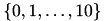is defined. Please note, that the index interval is closed on both ends, e.g. the first and the last index is given.

In addition to a standard index set, you may also define a stride, e.g. a distance between elements in the index set:

Range r2( 10, 20, 2 );

This defines the index set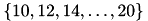For convenience, a constructor for an index set containing just one element is also available:

Range r3( 21 );

yields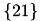.

# Vectors

## Construction

Usually, a BLAS vector is intialised with the corresponding size as the constructor argument:

Vector< double > x( 10 );

creates a double vector of size 10. Leaving out the size argument, intialises the vector with size 0:

Vector< double > y;

Providing another vector in the constructor, creates a new vector pointing to the same data as the argument vector:

Vector< double > z( x );

Changing z also changes x!

With the usage of range objects, parts of vectors can be represented as another vector:

Vector< double > x1( 10 );
Vector< double > x2( x1, Range( 5, 10 ) );
Vector< double > x3( x2, Range( 0, 5, 2 ) );

Here, x2 addresses the second half of the coefficients of x1 whereas x3 contains each second coefficient of x2 and by that, the coefficients 5, 7 and 9 of x1.

By default, the policy for the copy constructors is to use a reference to the given data. You may change that, such that a copy of the data is created, e.g. a new memory block is allocated:

Vector< double > x3( x2, Range( 0, 5, 2 ), copy_value );

Now, x3 does not address any coefficients in x1 or x2.

## Accessing Coefficients and Vector Properties

Single coefficients in vectors are accessed using the {()} operator:

Vector< double > x( 10 );
for ( idx_t i = 0; i < 10; ++i )
x( i ) = i;
for ( idx_t i = 0; i < 10; ++i )
std::cout << x( i ) << std::endl;

The length of a vector is accessible via the length() function:

for ( idx_t i = 0; i < x.length(); ++i )
x( i ) = i;

## Algebra

Most of the BLAS vector functions are available in HLIBpro and some, which have no representation in the BLAS package.

An examples, involving filling, scaling vectors, forming linear combinations and computing dot products:

Vector< double > x( 10 );
Vector< double > y( 10 );
Vector< double > z( 10 );
fill( 2, x ); // x is fill with 2
fill( 3.5, y ); // y is fill with 3.5
copy( x, z ); // copy coefficients of x to z
add( 0.5, y, z ); // set z ≔ z + 0.5 x
std::cout << dot( x, z ) << std::endl; // compute x^H·z

As mentioned before, you can not mix vectors of different value type, e.g.

Vector< double > x( 10 );
Vector< float > y( 10 );
copy( x, y ); // ERROR

since BLAS/LAPACK do not support such mixing

For a complete overview of the algebra functions refer to the BLAS module.

# Matrices

## Construction

Constructing matrices follows the same scheme as vectors, with the only difference being the additional dimension:

Matrix< double > M1( 10, 20 );
Matrix< complex > M2( 20, 20 );
Matrix< double > M3( M1 );
Matrix< double > M4;

Addressing subblocks of a given matrix is done by providing Range objects:

Matrix< double > M1( 20, 20 );
Matrix< double > M2( M1, Range( 10, 19 ), Range( 0, 19 ) );
Matrix< double > M3( M2, Range( 0, 9 ), Range( 0, 19, 2 ) );

Here, M2 references the last 10 rows of M1. M3 also shares these coefficients but further restricts the elements to each second column of M1. Hence

M1( 14, 10 ) = 2.0;
M2( 4, 10 ) = 3.0;
M3( 4, 5 ) = 4.0;

will affect the same memory position.

Remarks
This also introduces the coefficient access for matrices, again being the standard index operator.

The standard copy policy for matrices, i.e. copy_reference, can be changed by the last, optional parameter to the above constructor:

Matrix< double > M4( M2, Range( 0, 9 ), Range( 0, 19, 2 ), copy_value );

This will create a 10×10 matrix with coefficients copied from matrix M2.

## Algebra

As for vectors, the arithmetic functionality is implemented as functions and not as special C++ operators for objects of type Matrix.

### Matrix views

𝓗𝖫𝖨𝖡𝗉𝗋𝗈 provides different <emph>matrix view</emph> for accessing matrices, e.g. transposed and hermitian view. A view object will not change or copy the data of a matrix but changes for instance the way the data is access, e.g. interchanging the indices. For a given matrix

Matrix< double > M( 10, 10 );

The transposed view of M is defined by

transpose( M );

These views may be used for arithmetic functions, albeit not all functions, to simplify the usage and to enhance the performance of these functions.

### Matrix Matrix Arithmetic

The most common form of matrix vector arithmetic is the matrix vector multiplication in the general form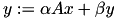:

Matrix< double > M( 20, 10 );
Vector< double > x( 10 );
Vector< double > y( 20 );
mulvec( 2.0, M, x, 1.0, y );

Using matrix views allows the multiplication with the transposed or adjoint matrix:

mulvec( 2.0, transposed(M), x, 1.0, y );
mulvec( 2.0, adjoint(M), x, 1.0, y );

If M is a triangular matrix, the multiplication is performed in place, i.e. the single argument vector is modified:The exact shape of the matrix is defined by additional arguments of type tri_type_t (lower_triangular or upper_triangular) and diag_type_t (unit_diag or general_diag). The second argument describes the diagonal which is either identical to 1 or not:

mulvec_tri( upper_triangular, general_diag, transposed(M), x );
Remarks
The shape of the matrix, e.g. lower or upper triangular, always refers to the original matrix and not the provided view!

Also available is the rank-1 update of a matrix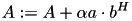:

Matrix< double > M( 20, 30 );
Vector< double > a( 20 );
Vector< double > b( 30 );
add_r1( 0.5, a, b, M );

For triangular matrices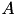, solving equation systems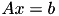is supported via:

Matrix< double > A( 20, 20 );
Vector< double > x( 20 );
solve_tri( lower_triangular, unit_diag, adjoint(A), x )

Here, upon input x contains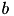b and is overwritten with the result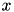.

### Matrix Matrix Arithmetic

The BLAS namespace defines common functions for single matrices and between matrices. Single matrix functions are

Matrix< complex > M( 20, 20 );
fill( 3.14, M ); // fill M with 3.14
scale( 2.72, M ); // scale M by 2.72
conj( M ); // replace coefficients with conjugate
transpose( M ); // inplace transpose or
conj_transpose( M ); // conjugate transpose of M
Remarks
PLease note, that the last two functions will only work for square matrices!

Different matrix norms are available:

std::cout << normF( M ) << std::endl; // print Frobenius norm
std::cout << norm2( M ) << std::endl; // print spectral norm
std::cout << cond( M ) << std::endl; // print condition number

Other functions, involving more matrices are:

Matrix< double > M1( 10, 20 );
Matrix< double > M2( 10, 20 );
Matrix< double > M3( 10, 10 );
Matrix< double > M4( 10, 10 );
prod( 1.0, M1, transposed( M2 ), 0.0, M3 );

Inversion and factorisation functions are:

invert( M ); // compute M^-1
lu( M ); // compute LU factorisation
ldlt( M ); // compute LDL^T factorisation
ldlh( M ); // compute LDL^H factorisation
llt( M ); // compute LL^T factorisation
llh( M ); // compute LL^H factorisation
qr( M, R ); // compute QR factorisation
Remarks
All factorisation functions are without pivoting!

It is also possible to solve matrix equations of the form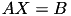with knownand:

Matrix< double > A( 10, 10 );
Matrix< double > X( 10, 10 );
solve( A, X );

X will initially contain the matrix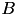. Furthermore, the content of the matrix A will be destroyed during computation.

Ifis a triangular matrix, the equation may take the more general forms: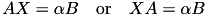The corresponding side from which to applyto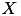is defined by a parameter (form_left or form_right):

Matrix< double > A( 10, 10 );
Matrix< double > X( 10, 5 );
solve_tri( from_left, upper_triangular, unit_diag, 1.0, A, X );

Eigenvalues and singular values and vectors of a matrix can also be computed:

Vector< double > eig_val;
Matrix< double > eig_vec;
eigen( M, eigval, eigvec ); // compute all eigen values/vectors of M
Vector< double > S;
Matrix< double > V;
svd( M, S, V ); // compute SVD M = USV^H (U overwrites M)
sv( M, S ); // compute singular values of M

If M is symmetric, selected eigen values may be computed:

eigen( M, B::Range( 0, 1 ), eigval, eigvec ); // compute first 2 eigen values/vectors